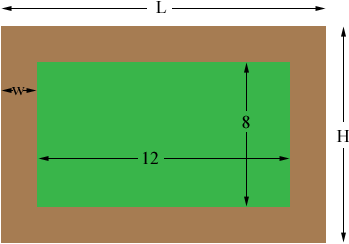SEARCH HOMEMath Central Quandaries & QueriesQuestion from Aniish, a student: A picture 8inches by 12inches is placed in a frame of uniform width. If the area of the frame equals the area of the picture find the width of the frame?Hi Aniish,

In my diagram the width of the frame is $w$ inches and the frame is $L$ inches long and $H$ inches wide.From the diagram $L = 12 + 2 \times w$ and $H = 8 + 2\times w.$ What is the area of the picture? What is the area of the picture and frame together? Subtract the area of the picture from the area of the picture and frame together to find the area of the frame. This equals the area of the picture so set them equal to obtain an equation that you can solve for $w.$

PennyMath Central is supported by the University of Regina and The Pacific Institute for the Mathematical Sciences.